## Whole Numbers and Place Value

### Learning Outcomes

• Identify whole numbers from a list of numbers
• Identify counting numbers from a list of numbers
• Model whole numbers
• Identify the place value of a digit

## Identify Counting Numbers and Whole Numbers

Learning algebra is similar to learning a language. You start with a basic vocabulary and then add to it as you go along. You need to practice often until the vocabulary becomes easy to you. The more you use the vocabulary, the more familiar it becomes.

Algebra uses numbers and symbols to represent words and ideas. Let’s look at the numbers first. The most basic numbers used in algebra are those we use to count objects: $1,2,3,4,5,\dots$ and so on. These are called the counting numbers. The notation “…” is called an ellipsis, which is another way to show “and so on,” or that the pattern continues endlessly. Counting numbers are also called natural numbers.

### Counting Numbers or Natural Numbers

The counting numbers start with $1$ and continue.
$1,2,3,4,5\dots$

Counting numbers and whole numbers can be visualized on a number line as shown below.

The numbers on the number line increase from left to right, and decrease from right to left.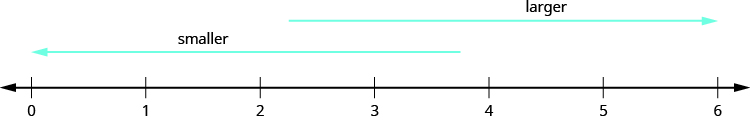The point labeled $0$ is called the origin. The points are equally spaced to the right of $0$ and labeled with the counting numbers. When a number is paired with a point, it is called the coordinate of the point.

The discovery of the number zero was a big step in the history of mathematics. Including zero with the counting numbers gives a new set of numbers called the whole numbers.

### Whole Numbers

The whole numbers are the counting numbers and zero.
$0,1,2,3,4,5\ldots$

We stopped at $5$ when listing the first few counting numbers and whole numbers. We could have written more numbers if they were needed to make the patterns clear.

### example

Which of the following are

$1$. counting numbers?

$2$. whole numbers?

$0,\frac{1}{4},3,5.2,15,105$

Solution

1. The counting numbers start at $1$, so $0$ is not a counting number. The numbers $3,15,\text{and }105$ are all counting numbers.
2. Whole numbers are counting numbers and $0$. The numbers $0,3,15,\text{and }105$ are whole numbers.

The numbers $\frac{1}{4}$ and $5.2$ are neither counting numbers nor whole numbers. We will discuss these numbers later.

## Model Whole Numbers

Our number system is called a place value system because the value of a digit depends on its position, or place, in a number. The number $537$ has a different value than the number $735$. Even though they use the same digits, their value is different because of the different placement of the $3$ and the $7$ and the $5$.

Money gives us a familiar model of place value. Suppose a wallet contains three $100 bills, seven$10 bills, and four $1 bills. The amounts are summarized in the image below. How much money is in the wallet?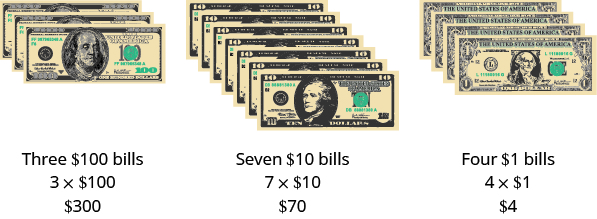Find the total value of each kind of bill, and then add to find the total. The wallet contains$374.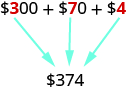Base-10 blocks provide another way to model place value, as shown in the image below. The blocks can be used to represent hundreds, tens, and ones. Notice that the tens rod is made up of $10$ ones, and the hundreds square (also known as a “flat”) is made of $10$ tens, or $100$ ones.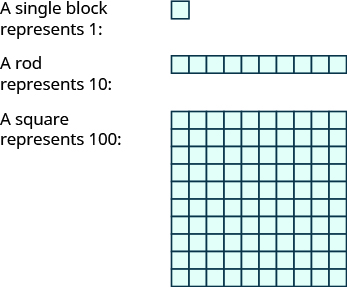The image below shows the number $138$ modeled with base-10 blocks.

We use place value notation to show the value of the number $138$.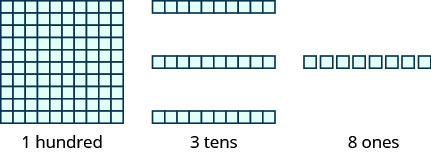Digit Place value Number Value Total value
$1$ hundreds $1$ $100$ $100\phantom{\rule{1 em}{0ex}}$
$3$ tens $3$ $10$ $30\phantom{\rule{1 em}{0ex}}$
$8$ ones $8$ $1$ $+\phantom{\rule{.5 em}{0ex}}8\phantom{\rule{1 em}{0ex}}$
$\text{Sum =}138\phantom{\rule{1 em}{0ex}}$

### example

Use place value notation to find the value of the number modeled by the base-10 blocks shown.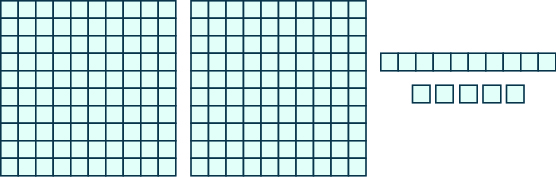### try it

Use place value notation to find the value of the number modeled by the base-10 blocks shown.## Identify the Place Value of a Digit

By looking at money and base-10 blocks, we saw that each place in a number has a different value. A place value chart is a useful way to summarize this information. The place values are separated into groups of three, called periods. The periods are ones, thousands, millions, billions, trillions, and so on. In a written number, commas separate the periods.

Just as with the $\text{base - 10}$ blocks, where the value of the tens rod is ten times the value of the ones block and the value of the hundreds square is ten times the tens rod, the value of each place in the place-value chart is ten times the value of the place to the right of it.
The chart below shows how the number $5,278,194$ is written in a place value chart.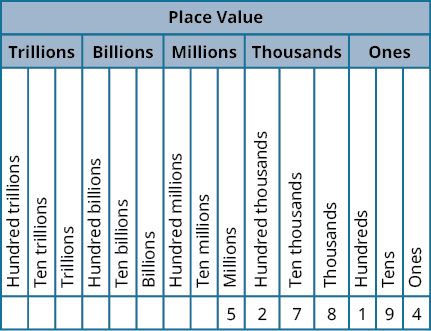• The digit $5$ is in the millions place. Its value is $5,000,000$.
• The digit $2$ is in the hundred thousands place. Its value is $200,000$.
• The digit $7$ is in the ten thousands place. Its value is $70,000$.
• The digit $8$ is in the thousands place. Its value is $8,000$.
• The digit $1$ is in the hundreds place. Its value is $100$.
• The digit $9$ is in the tens place. Its value is $90$.
• The digit $4$ is in the ones place. Its value is $4$.

### example

In the number $63,407,218$; find the place value of each of the following digits:

1. $7$
2. $0$
3. $1$
4. $6$
5. $3$

### try it

The video below shows more examples of how to determine the place value of a digit in a number.

## Contribute!

Did you have an idea for improving this content? We’d love your input.